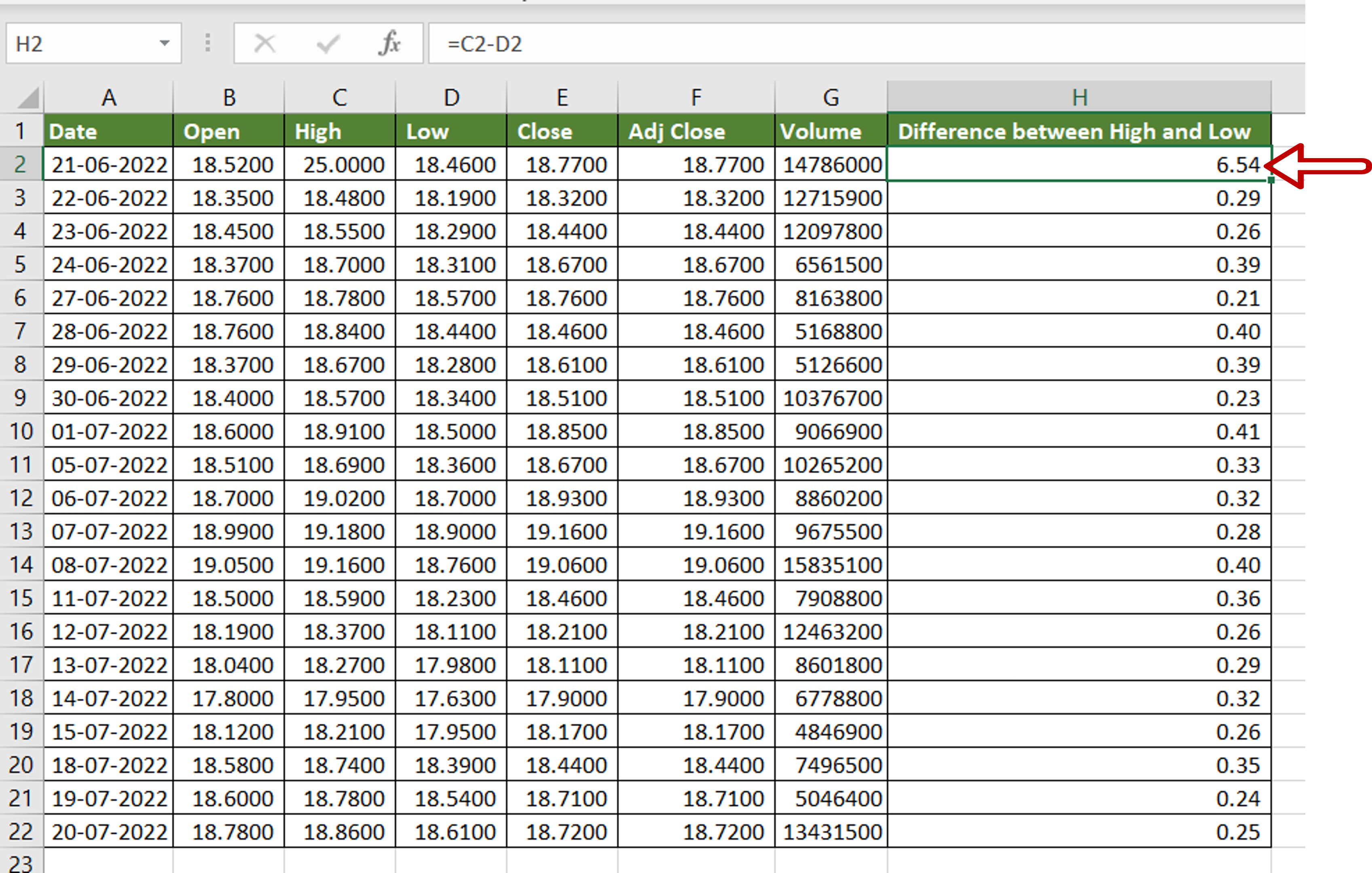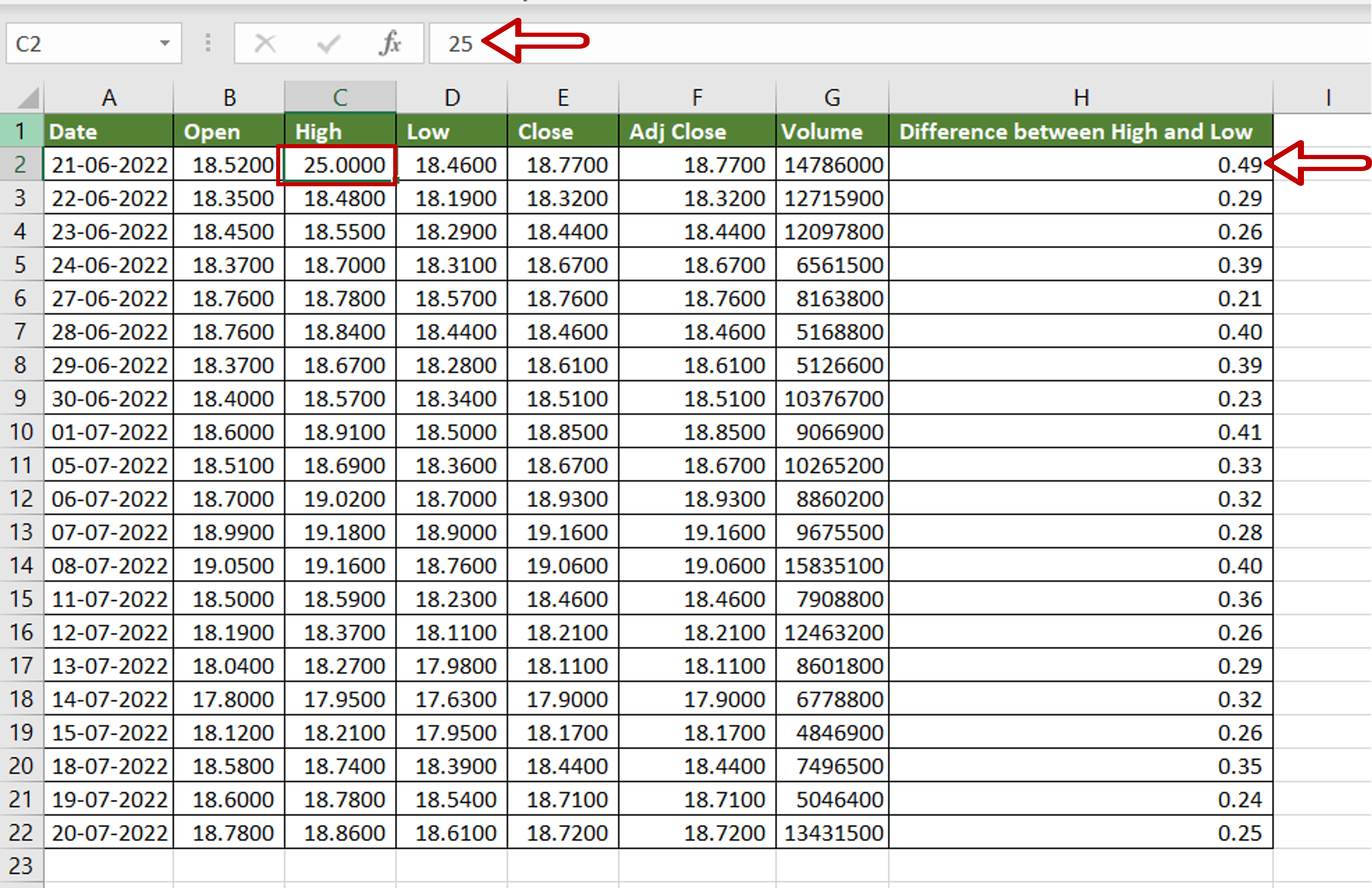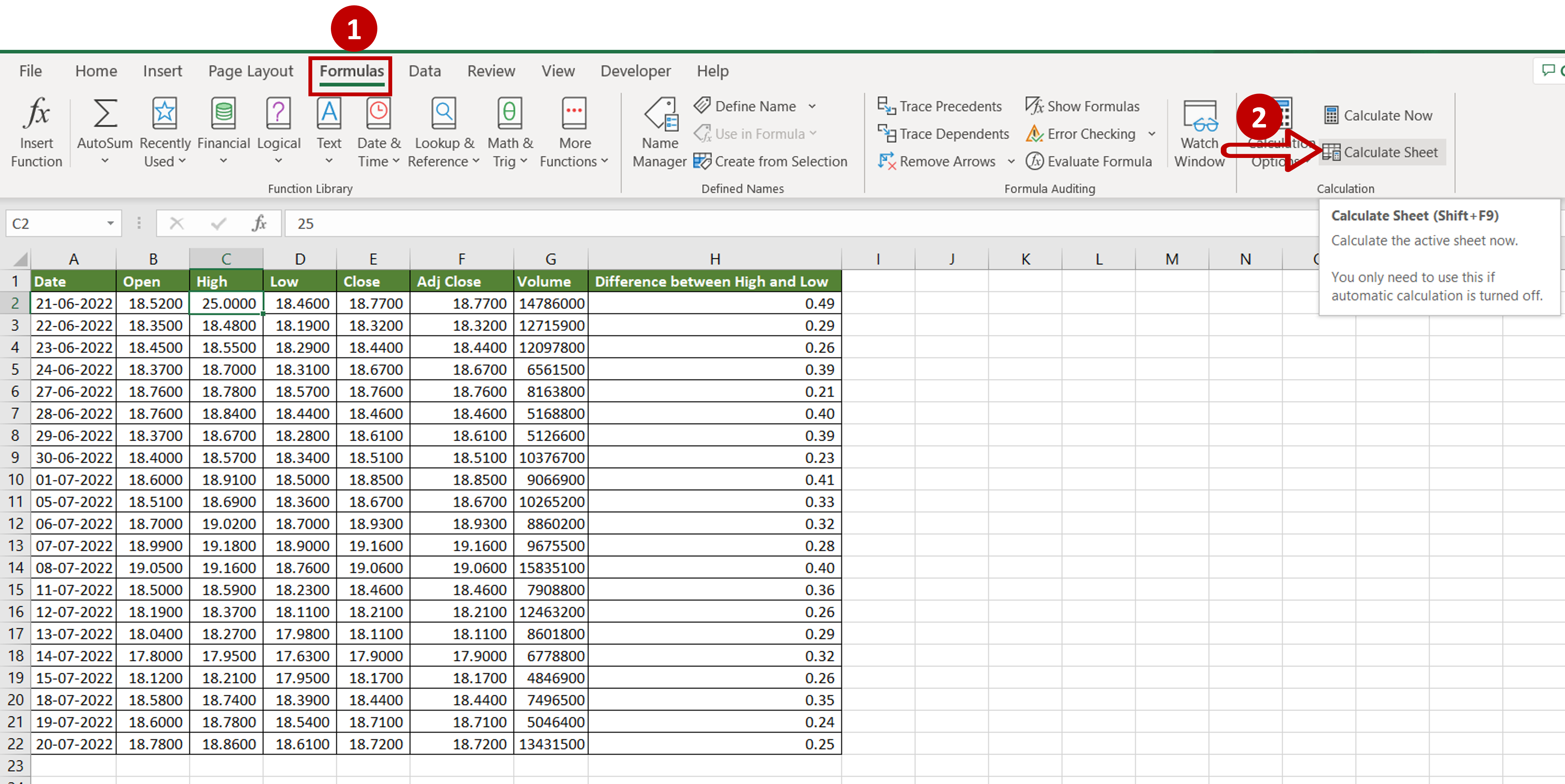# How to recalculate in Excel

You can watch a video tutorial here.One of the great things about Excel is the ability to create formulas that use cell references instead of actual values. Using cell references in formulas introduces a lot of flexibility in calculations. Values in the source columns can be changed and the calculated columns will automatically be updated. When you have a large worksheet in which there are many complex formulas, you may need to turn off the default setting as the automatic recalculation takes time. By setting the calculation option to ‘Manual’, you can control when the recalculation is to be done.

### Step 1 – Update the data– Change the first value in the ‘High’ column
– The formula in the ‘Difference between High and Low’ column does not change

### Step 2 – Recalculate the sheet– Go to Formulas > Calculation
– Click on Calculate Sheet or Press Shift+F9
Note: The Calculate Now button recalculates all the sheets in the active workbook

### Step 3 – Check the result– The sheet is recalculated and the value is updated